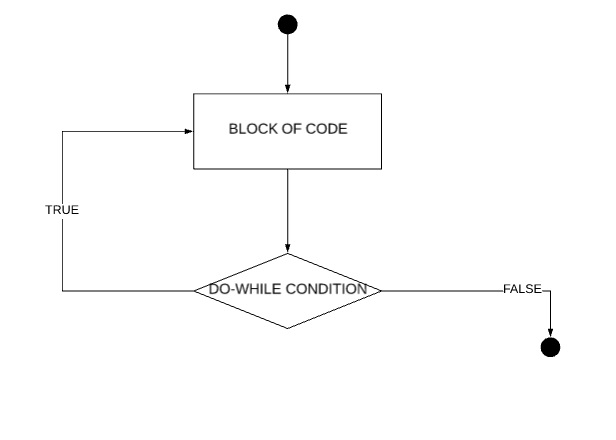# The do...while loop in Scala

The do...while loop in Scala is used to run a block of code multiple time until a specific condition is true. In this tutorial, we will learn about the do...while loop its use, syntax, example.
Submitted by Shivang Yadav, on June 23, 2019

## do...while loop in Scala

do...while loop in Scala is used to run a block of code multiple numbers of time. The number of executions is defined by an exit condition. If this condition is TRUE the code will run otherwise it runs the first time only

The do...while loop is used when the program does not have information about the exact number of executions taking place. The number of executions is defined by an exit condition that can be any variable or expression, the value evaluated in TRUE if it's positive and FALSE if it's zero.

This loop always runs once in the life span of code. If the condition is initially FALSE. The loop will run once in this case.

The do...while loop is also called exit controlled loop because its condition is checked after the execution of the loop's code block.

Syntax of do...while loop:

```    do{
//Code to be executed...
}
while(condition);
```

Flow chart of do...while loop:Example of do...while loop:

```object MyClass {
def main(args: Array[String]) {
var myVar = 12;
println("This code prints myVar even if it is greater that 10")
do{
println(myVar)
myVar += 2;
}
while(myVar <= 10)
}
}
```

Output

```This code prints myVar even if it is greater that 10
12
```

Code explanation:

This code implements the use of the do...while loop in Scala. The do...while loop being an exit control loop checks the condition after the first run. This is why the code prints 12 but the condition is myVar should not be greater than 10. In this we put the condition after the code block this means the code will run like this, print myVar increment it by 2 (using assignment operator) and then checks for the condition of the loop.

The assignments for the do...while loop that you can complete and submit to know your progress.

Assignment 1 (difficulty - beginner): Print all prime numbers from 342 - 422 that is divisible by 3. (use do-while loop and functions.)

Assignment 2 (difficulty - intermediate): Print all number between 54 - 1145 that have 11,13 and 17 as a factor.

Top MCQs